# 用TensorFlow和TensorBoard从零开始构建ConvNet（CNN）

+关注继续查看

1.选择数据集

2.选择一个模型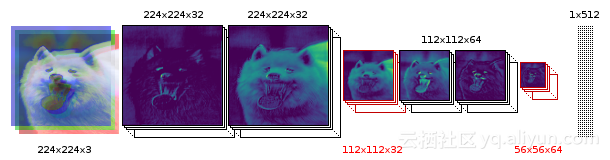3.处理和构建数据集：

import imFunctions as imf
import tensorflow as tf
import scipy.ndimage
from scipy.misc import imsave
import matplotlib.pyplot as plt
import numpy as np


imf.downloadImages('annotations.tar.gz', 19173078)
imf.maybeExtract('annotations.tar.gz')
imf.maybeExtract('images.tar.gz')


imf.sortImages(0.15)

train_x, train_y, test_x, test_y, classes, classLabels = imf.buildDataset()

4.如何卷积实现功能

1.计算给定4-D输入和滤波张量的2-D卷积。

2.给定一个形状为[batchin_heightin_widthin_channels]的输入张量以及形状为[filter_heightfilter_widthin_channelsout_channels]的过滤器/内核张量。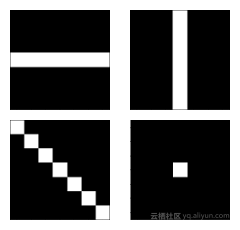gray = np.mean(image,-1)
X = tf.placeholder(tf.float32, shape=(None, 224, 224, 1))
conv = tf.nn.conv2d(X, filters, [1,1,1,1], padding="SAME")
test = tf.Session()
test.run(tf.global_variables_initializer())
filteredImage = test.run(conv, feed_dict={X: gray.reshape(1,224,224,1)})
tf.reset_default_graph()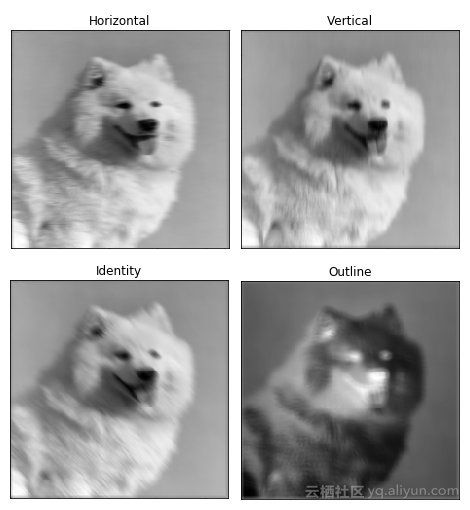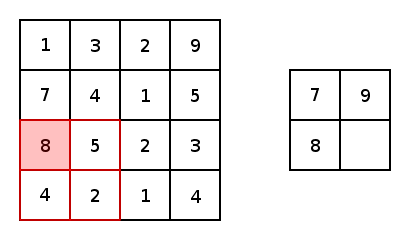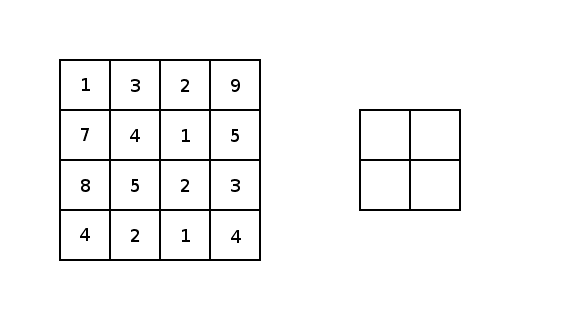5．创建ConvNet

X = tf.placeholder(tf.float32, shape=(None, 224, 224, 3))
Y_ = tf.placeholder(tf.float32, [None, classes])
keepRate1 = tf.placeholder(tf.float32)
keepRate2 = tf.placeholder(tf.float32)


# CONVOLUTION 1 - 1
with tf.name_scope('conv1_1'):
filter1_1 = tf.Variable(tf.truncated_normal([3, 3, 3, 32], dtype=tf.float32,stddev=1e-1), name='weights1_1')
stride = [1,1,1,1]
conv = tf.nn.conv2d(X, filter1_1, stride, padding='SAME')
biases = tf.Variable(tf.constant(0.0, shape=, dtype=tf.float32)，trainable=True, name='biases1_1')
conv1_1 = tf.nn.relu(out)


# CONVOLUTION 1 - 2
with tf.name_scope('conv1_2'):
filter1_2 = tf.Variable(tf.truncated_normal([3, 3, 32, 32], dtype=tf.float32,stddev=1e-1), name='weights1_2')
conv = tf.nn.conv2d(conv1_1, filter1_2, [1,1,1,1], padding='SAME')
biases = tf.Variable(tf.constant(0.0, shape=, dtype=tf.float32),trainable=True, name='biases1_2')
conv1_2 = tf.nn.relu(out)


# POOL 1
with tf.name_scope('pool1'):
pool1_1 = tf.nn.max_pool(conv1_2,
ksize=[1, 2, 2, 1],
strides=[1, 2, 2, 1],
name='pool1_1')
pool1_1_drop = tf.nn.dropout(pool1_1, keepRate1)


# CONVOLUTION 2 - 1
with tf.name_scope('conv2_1'):
filter2_1 = tf.Variable(tf.truncated_normal([3, 3, 32, 64], dtype=tf.float32,stddev=1e-1), name='weights2_1')
conv = tf.nn.conv2d(pool1_1_drop, filter2_1, [1, 1, 1, 1], padding='SAME')
biases = tf.Variable(tf.constant(0.0, shape=, dtype=tf.float32),trainable=True, name='biases2_1')
conv2_1 = tf.nn.relu(out)
# CONVOLUTION 2 - 2
with tf.name_scope('conv2_2'):
filter2_2 = tf.Variable(tf.truncated_normal([3, 3, 64, 64], dtype=tf.float32,stddev=1e-1), name='weights2_2')
conv = tf.nn.conv2d(conv2_1, filter2_2, [1, 1, 1, 1], padding='SAME')
biases = tf.Variable(tf.constant(0.0, shape=, dtype=tf.float32),trainable=True, name='biases2_2')
conv2_2 = tf.nn.relu(out)
# POOL 2
with tf.name_scope('pool2'):
pool2_1 = tf.nn.max_pool(conv2_2,
ksize=[1, 2, 2, 1],
strides=[1, 2, 2, 1],
name='pool2_1')
pool2_1_drop = tf.nn.dropout(pool2_1, keepRate1)


with tf.name_scope('fc1') as scope:
shape = int(np.prod(pool2_1_drop.get_shape()[1:]))
fc1w = tf.Variable(tf.truncated_normal([shape, 512], dtype=tf.float32,stddev=1e-1), name='weights3_1')
fc1b = tf.Variable(tf.constant(1.0, shape=, dtype=tf.float32),trainable=True, name='biases3_1')
pool2_flat = tf.reshape(pool2_1_drop, [-1, shape])
fc1 = tf.nn.relu(out)
fc1_drop = tf.nn.dropout(fc1, keepRate2)


#FULLY CONNECTED 3 & SOFTMAX OUTPUT
with tf.name_scope('softmax') as scope:
fc2w = tf.Variable(tf.truncated_normal([512, classes], dtype=tf.float32,stddev=1e-1), name='weights3_2')
fc2b = tf.Variable(tf.constant(1.0, shape=[classes], dtype=tf.float32),trainable=True, name='biases3_2')
Y = tf.nn.softmax(Ylogits)


6.创建损失函数和优化器:

numEpochs = 400
batchSize = 10
alpha = 1e-5


with tf.name_scope('cross_entropy'):
cross_entropy = tf.nn.softmax_cross_entropy_with_logits(logits=Ylogits, labels=Y_)
loss = tf.reduce_mean(cross_entropy)
with tf.name_scope('accuracy'):
correct_prediction = tf.equal(tf.argmax(Y, 1), tf.argmax(Y_, 1))
accuracy = tf.reduce_mean(tf.cast(correct_prediction, tf.float32))
with tf.name_scope('train'):


sess = tf.Session()
init = tf.global_variables_initializer()
sess.run(init)


7. 准备TensorBoard

writer_1 = tf.summary.FileWriter("/tmp/cnn/train")
writer_2 = tf.summary.FileWriter("/tmp/cnn/test")
tf.summary.scalar('Loss', loss)
tf.summary.scalar('Accuracy', accuracy)
tf.summary.histogram("weights1_1", filter1_1)
write_op = tf.summary.merge_all()


8.训练模型

steps = int(train_x.shape/batchSize)
for i in range(numEpochs):
accHist = []
accHist2 = []
train_x, train_y = imf.shuffle(train_x, train_y)
for ii in range(steps):
#Calculate our current step
step = i * steps + ii
acc = sess.run([accuracy], feed_dict={X: train_x[(ii*batchSize):((ii+1)*batchSize),:,:,:], Y_: train_y[(ii*batchSize):((ii+1)*batchSize)], keepRate1: 1, keepRate2: 1})
accHist.append(acc)
if step % 5 == 0:
# Get Train Summary for one batch and add summary to TensorBoard
summary = sess.run(write_op, feed_dict={X: train_x[(ii*batchSize):((ii+1)*batchSize),:,:,:], Y_: train_y[(ii*batchSize):((ii+1)*batchSize)], keepRate1: 1, keepRate2: 1})
writer_1.flush()

# Get Test Summary on random 10 test images and add summary to TensorBoard
test_x, test_y = imf.shuffle(test_x, test_y)
summary = sess.run(write_op, feed_dict={X: test_x[0:10,:,:,:], Y_: test_y[0:10], keepRate1: 1, keepRate2: 1})
writer_2.flush()
sess.run(train_step, feed_dict={X: train_x[(ii*batchSize):((ii+1)*batchSize),:,:,:], Y_: train_y[(ii*batchSize):((ii+1)*batchSize)], keepRate1: 0.2, keepRate2: 0.5})


9.可视化

tensorboard --logdir="/tmp/cnn/"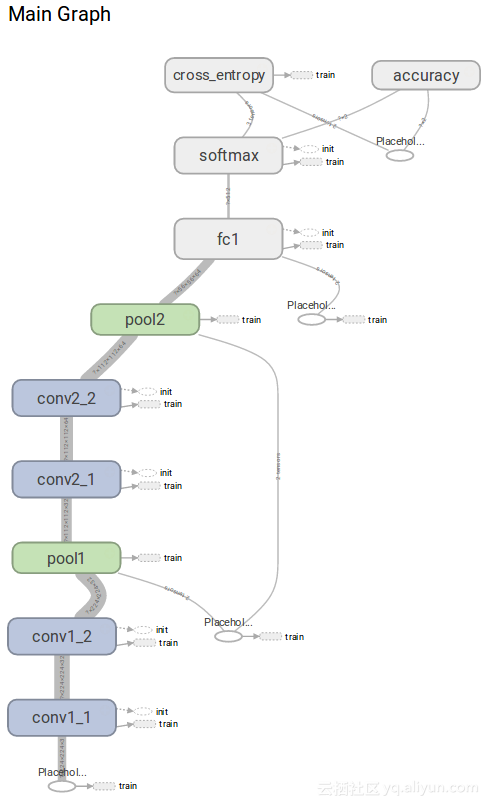10.性能测量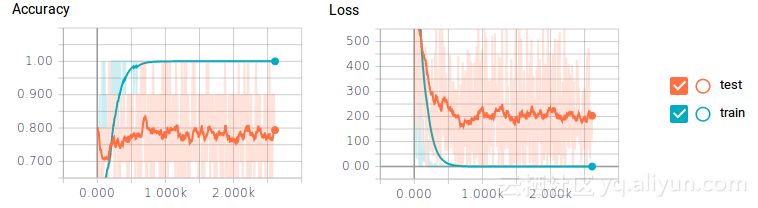4.可视化进化过滤器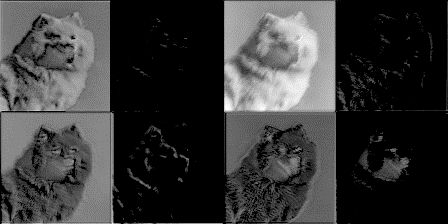Justin Francis目前是加拿大阿尔伯塔大学的本科生。贾斯汀还在大学工程俱乐部自主机器人车辆项目arvp.org）的软件团队中帮助实施和实验了深入学习和强化学习算法1081 04054 04030 0ASP.NET Core MVC中构建Web API

888 0908 04397 02862 0Linux上TensorFlow的深入研究：构建一个低成本、快速、精准的图像分类器
1552 02023 0829 0
【方向】

696

5

《SaaS模式云原生数据仓库应用场景实践》

《看见新力量：二》电子书